# Working backwards from the Area Under a Curve?

• I
• mikejm
AUC" and..."c"?There is not a line of best fit that would give a good approximation of the relationship between "AUC" and "c". However, you could use a root-finding method (like Newton-Raphson perhaps) to find an approximate value for c. To do that you could rewrite the equation as (1-c)AUC - (100,000,000^(1-c) - 1) = 0 and use the root-finding method to find a value of c that makes the left

#### mikejm

I am working on a synthesizer project and have reached a point that I am stumped on.

I am in this part trying to work from a basic curve of y=1/x^c (where x≥1):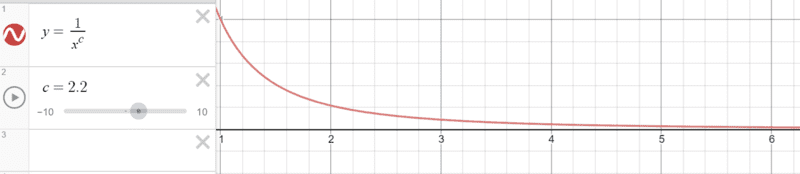As I understand, area under the curve between x=1 and x=100,000,000 (ie. more than I need for a rough approximation) would be: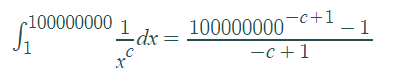So essentially,

AUC = (100,000,000^(-c+1) - 1)/(-c+1)

Can this equation be re-arranged to roughly approximate for "c="?

I would like to be able to give an "AUC" level between an arbitrary range, and from each AUC level given, calculate approximately "c". This "c" will then be put back into the original y=1/x^c equation. The shape of the y=1/x^c curve will then dictate a part of the synth's sound.

The point is to be able to adjust the y=1/x^c curve with a knob based on "AUC" rather than directly based on "c", as "AUC" makes more sense in this case.

Is this possible to do even approximately in this way?

Thanks a lot,
Mike

#### Attachments

Last edited:
mikejm said:
I am working on a synthesizer project and have reached a point with some math to be solved that I am stumped on.

I am in this part trying to work from a basic curve of y=1/x^c (where x≥1):View attachment 226125

As I understand, area under the curve between x=1 and x=100,000,000 (ie. more than I need for a rough approximation) would be:
View attachment 226126

So essentially,

AUC = (100,000,000^(-c+1) - 1)/(-c+1)

I would like to be able to give an "AUC" level between an arbitrary range, and from each AUC level given, calculate approximately "c". This "c" will then be put back into the original y=1/x^c equation. The shape of the y=1/x^c curve will then dictate a part of the synth's sound.

The point is to be able to adjust the y=1/x^c curve with a knob based on "AUC" rather than directly based on "c", as "AUC" makes more sense in this case.

Is this possible to do even approximately in this way?

Thanks a lot,
Mike
If you are asking if there is some way to solve the equation AUC = (100,000,000^(-c+1) - 1)/(-c+1) for c, then the answer is no. However, you can use a root-finding method (like Newton-Raphson perhaps) to find an approximate value for c. To do that you could rewrite the equation as (1-c)AUC - (100,000,000^(1-c) - 1) = 0 and use the root-finding method to find a value of c that makes the left hand side zero.

tnich said:
If you are asking if there is some way to solve the equation AUC = (100,000,000^(-c+1) - 1)/(-c+1) for c, then the answer is no. However, you can use a root-finding method (like Newton-Raphson perhaps) to find an approximate value for c. To do that you could rewrite the equation as (1-c)AUC - (100,000,000^(1-c) - 1) = 0 and use the root-finding method to find a value of c that makes the left hand side zero.

Thanks for clarifying tnich! Looks like that type of math would be very over my head and excessively burdensome to be doing continuously on an ongoing basis for my synth.

What about a simpler way? If I can work out a table of relationships between "AUC" and "c", should I not be able to find a line of best fit that would give a good approximation of the relationship?

I tried to start doing that here: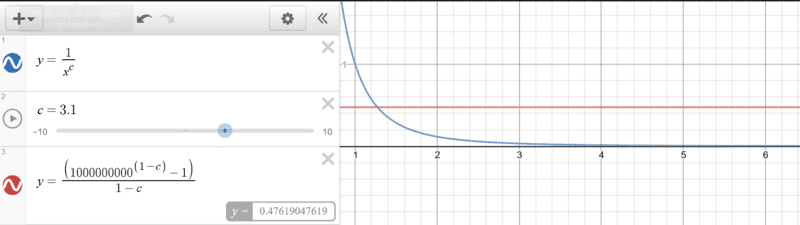Which lead to this table and graph of AUC ~ c: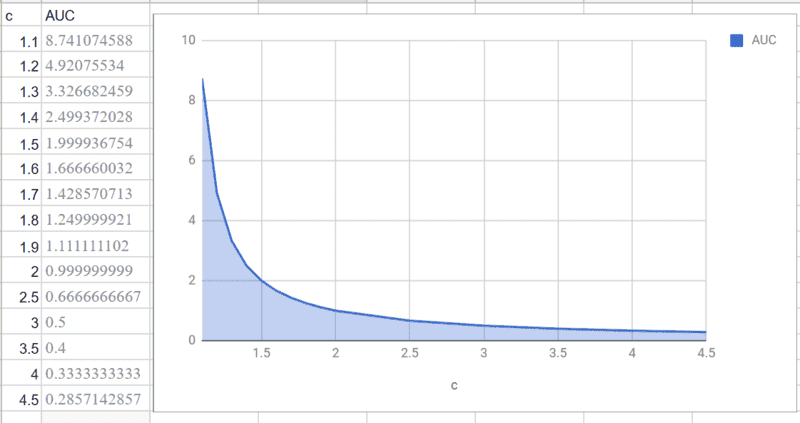Is there any way to get a good approximate equation to describe the relationship from this or an expanded data set? How would I get that?

If not, worse case scenario, I can set up a table with 500 such values or so, and use that as a "reference" for the conversion when needed, but that's less favorable.

Thanks again!

#### Attachments

Last edited:
mikejm said:
Thanks for clarifying tnich! Looks like that type of math would be very over my head and excessively burdensome to be doing continuously on an ongoing basis for my synth.

What about a simpler way? If I can work out a table of relationships between "AUC" and "c", should I not be able to find a line of best fit that would give a good approximation of the relationship?

I tried to start doing that here:

View attachment 226128

Which lead to this table and graph of AUC ~ c:

View attachment 226129
mikejm said:
Thanks for clarifying tnich! Looks like that type of math would be very over my head and excessively burdensome to be doing continuously on an ongoing basis for my synth.

What about a simpler way? If I can work out a table of relationships between "AUC" and "c", should I not be able to find a line of best fit that would give a good approximation of the relationship?

I tried to start doing that here:

View attachment 226128

Which lead to this table and graph of AUC ~ c:

View attachment 226129

Is there any way to get a good approximate equation to describe the relationship from this or an expanded data set? How would I get that?

Thanks again!

Is there any way to get a good approximate equation to describe the relationship from this or an expanded data set? How would I get that?

Thanks again!
For AUC < 9 a pretty good approximation is c = 1 + 1/AUC.

tnich said:
For AUC < 9 a pretty good approximation is c = 1 + 1/AUC.
You changed your upper limit of integration from ##10^8## to ##10^9##. Did you mean to?

tnich said:
You changed your upper limit of integration from ##10^8## to ##10^9##. Did you mean to?
Haha good eye. No I didn't mean to. Good eye. I don't think it will make a significant difference either way at that level though. I'll have to be more careful overall though.

tnich said:
For AUC < 9 a pretty good approximation is c = 1 + 1/AUC.

That looks perfect actually! For all readings where c>=1.3 it lines up beautifully to at least two decimal places with rounding. This is more than good enough. I will try it with AUC max setting of 3.333 (c>=1.3) and see what happens.

Thanks!

Unfortunately, I dug into my synth and I actually most typically need "c" values in the 0.7-0.8 range, which looks like a much crazier range: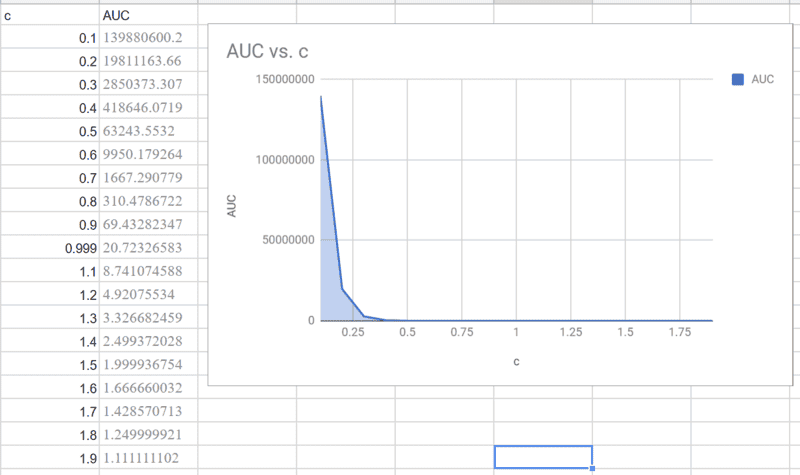But I also seem to have found a more ideal solution via mycurvefit.com:

Here is their 4 PL logistic fit model with c data for c=0.5-0.9 where they are able to get a perfect fit with not too complicated an equation: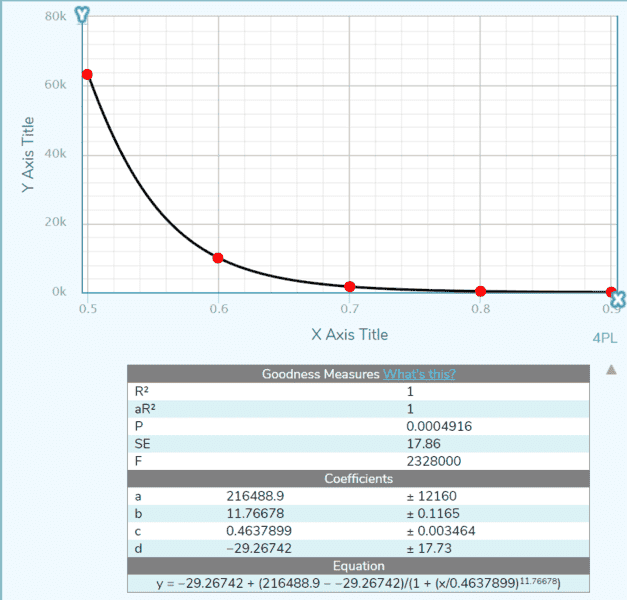Here is their 5 PL logistic fit with the full data from 0.1 to 0.9, again matching all points well, and creating what appears to be a good curve.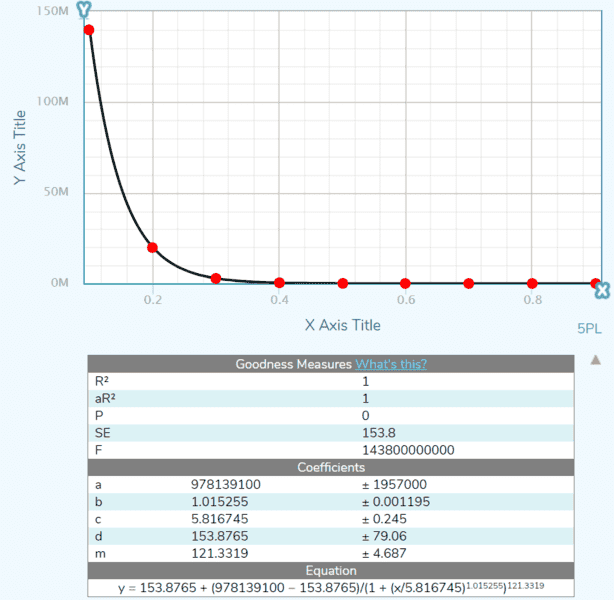If I redo these graphs with the x & y swapped, it will give me an equation that represents c = ___ * AUC ... which is exactly what I need.

So looks like the best solution is sampling some data and plugging it into this fit caclulator.

Thanks for the help! Does that make sense? Any diff between 5PL and 4PL If I want accuracy? Both look good so far.

(Awesome...)

#### Attachments

Last edited:
mikejm said:
Unfortunately, I dug into my synth and I actually most typically need "c" values in the 0.7-0.8 range, which looks like a much crazier range:

View attachment 226130

But I also seem to have found a more ideal solution via mycurvefit.com:

Here is their 4 PL logistic fit model with c data for c=0.5-0.9 where they are able to get a perfect fit with not too complicated an equation:

View attachment 226131

Here is their 5 PL logistic fit with the full data from 0.1 to 0.9, again matching all points well, and creating what appears to be a good curve.
View attachment 226132

If I redo these graphs with the x & y swapped, it will give me an equation that represents c = ___ * AUC ... which is exactly what I need.

So looks like the best solution is sampling some data and plugging it into this fit caclulator.

Thanks for the help! Does that make sense? Any diff between 5PL and 4PL If I want accuracy? Both look good so far.

(Awesome...)
Looks like a good approach, but that standard error (SE) is about the same size as the values you are trying to approximate. The graph is a little deceptive because of the scale (in millions). The AUC values in the 0.6 to 0.9 range all look like zero and you can't really tell if you matched them well or not. If you are not interested in values of c less than say 0.5, I suggest that you try leaving those out of your curve fit to get a better fit for the values you do care about.